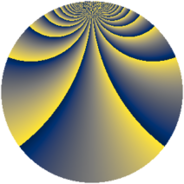# Properties

 Label 1380.2.baLevel $1380$ Weight $2$ Character orbit 1380.ba Rep. character $\chi_{1380}(59,\cdot)$ Character field $\Q(\zeta_{22})$ Dimension $2800$ Sturm bound $576$

# Related objects

## Defining parameters

 Level: $$N$$ $$=$$ $$1380 = 2^{2} \cdot 3 \cdot 5 \cdot 23$$ Weight: $$k$$ $$=$$ $$2$$ Character orbit: $$[\chi]$$ $$=$$ 1380.ba (of order $$22$$ and degree $$10$$) Character conductor: $$\operatorname{cond}(\chi)$$ $$=$$ $$1380$$ Character field: $$\Q(\zeta_{22})$$ Sturm bound: $$576$$

## Dimensions

The following table gives the dimensions of various subspaces of $$M_{2}(1380, [\chi])$$.

Total New Old
Modular forms 2960 2960 0
Cusp forms 2800 2800 0
Eisenstein series 160 160 0

## Trace form

 $$2800q - 32q^{4} - 26q^{6} - 36q^{9} + O(q^{10})$$ $$2800q - 32q^{4} - 26q^{6} - 36q^{9} - 10q^{10} - 32q^{16} - 4q^{21} - 52q^{24} - 36q^{25} - 17q^{30} - 62q^{34} - 2q^{36} - 34q^{40} - 84q^{45} - 40q^{46} - 304q^{49} + 26q^{54} + 13q^{60} - 104q^{61} - 8q^{64} - 40q^{66} - 72q^{69} - 36q^{70} - 92q^{76} + 68q^{81} + 38q^{84} + 20q^{85} + 198q^{90} - 4q^{94} - 372q^{96} + O(q^{100})$$

## Decomposition of $$S_{2}^{\mathrm{new}}(1380, [\chi])$$ into newform subspaces

The newforms in this space have not yet been added to the LMFDB.